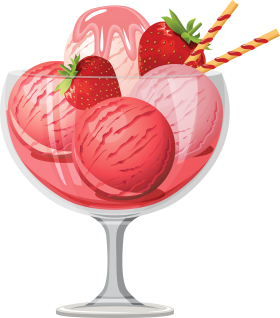# Consumer Equilibrium In Case of a Single Commodity

## Consumer Equilibrium

The state of balance obtained by an end-user of products refers to the number of goods and services they can buy, given their existing level of income and the prevailing level of cost prices. Consumer equilibrium permits a customer to get the most satisfaction possible from their income.

Related link: Theory Of Consumer Behaviour

(A) Meaning of consumer’s equilibrium Consumer’s Equilibrium means a state of maximum satisfaction.

A situation where a consumer spends his given income purchasing one or more commodities so that he gets maximum satisfaction and has no urge to change this level of consumption, given the prices of commodities, is known as the consumer’s equilibrium.

(B) Condition of consumer equilibrium in case of a single commodity

The consumer will be in the state of equilibrium when the following condition is fulfilled:

The marginal utility of commodity X in terms of rupees is equal to the price of commodity X in rupees. [MUx (in ) = Px (in )]

Or

Mux (in utils) = Px (in ) or MU of Commodity X (in utils) = Px (in

)

MUm (in utils) MU of Money (₹)(in utils)

(C) Hypothetical Schedule/ Numerical Example Let us take the example of a fruit ice cream. The price of an ice cream scoop is ₹30 and MUm, i.e., MU of money (₹1) = 1 util

Units ConsumedMU of ice -cream scoop  (in utils) {a}

 MU of money (Re. 1) (in utils) {b}

 MU of  ice -cream scoop  (in ₹) = {a}/ {b}

 Price of ice -cream scoop (₹)
1

2

3

4

5

50

40

30

20

10

1

1

1

1

1

50

40

30

20

10

>

>

=

<

<

30

30

30

30

30

(D) Explanation and conclusion In the given example, the level of consumer’s equilibrium is 3 units.

Where,

MU of ice cream in rupees = Price of ice cream in rupees, i.e., ₹30

• Before this level, e., at the first and the second level, MU > Price, i.e., benefit is more than cost. So, the consumer increases the consumption to attain equilibrium.
• After this level, i.e., at the fourth and the fifth level, MU < Price, e., benefit is less than cost. So, the consumer cuts or decreases the consumption to be in the state of equilibrium. Only at the level of 3 units, the condition of consumer’s equilibrium is fulfilled.

A consumer consumes the quantity at which MUx = Px to be in the state of equilibrium.

 Important Topic in Commerce:

### Lets Practice

1. If MUx> Px, the consumer ____________

A. Reaches the equilibrium

B. Starts incurring losses

C. Consumes more of X

D. Stops consuming X

Answer: C. Consumes more of X

2. A consumer gets maximum satisfaction when the ____________are the same.

A. Total utility and marginal utility

B. Prices of commodity and marginal utility

C. Price of commodity and total utility

D. Both (a) and (b)

Answer: B. Price of commodity and marginal utility

3.Equilibrium means the state of maximum _____________ ?